JKQTPlotter an extensive Qt4/Qt5 Plotter framework (including a fast variant and a LaTeX equation renderer!), written fully in C/C++ and without external dependencies
Example & Tutorial Projects

This section assembles some simple examples of usage. You can find more (complex) examples for the classes in this repository in the subfolder "test". All test-projects are Qt-projects that use qmake to build. You can load them into QtCreator easily.

# Overview of Examples&Tutorials

## Different Plot Data Styles

ScreenshotDescriptionNotesExample (JKQTPlotter): Very simple line-graph `JKQTPXYLineGraph`
C++-style QVector arrays of data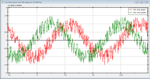Example (JKQTPlotter): Simple line-graph with live-data (speed test) `JKQTPXYLineGraph`
external `std::array<double,N>` data, not owned by datastore
live-data, measure plotting speed
tipps to increas plotting speed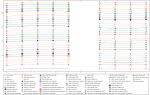Example (JKQTPlotter): Line Graph with Different Symbols and Line Styles `JKQTPXYLineGraph`
C++ vector of data
setting line styles and symbol styles
automatic graph coloring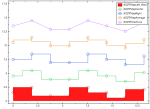Example (JKQTPlotter): Step Line Plots in Different Styles `JKQTPSpecialLineHorizontalGraph`
C++ vector of data
different step/special line modes, filled and line-only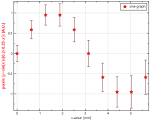Example (JKQTPlotter): Simple line-graph with error bars `JKQTPXYLineErrorGraph`
C-style arrays of data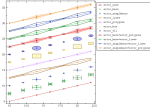Example (JKQTPlotter): Different Types of Errorindicators `JKQTPXYLineErrorGraph`
different styles of error indicators for x- and y-errors
C++-style QVector for data
styling error indicators
moving key and formatting plotter grid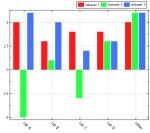Example (JKQTPlotter): Simple barchart `JKQTPBarVerticalGraph`
C-style arrays of dataExample (JKQTPlotter): Simple stacked barchart `JKQTPBarVerticalStackableGraph`, `JKQTPBarHorizontalStackableGraph`
C++-style vectors of data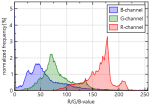Example (JKQTPlotter): Filled Curve Plots `JKQTPBarVerticalGraph`
setting/altering data in `JKQTPDatstore` directly
transparent plots
calculating histograms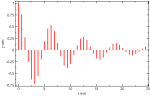Example (JKQTPlotter): Simple impulse plots `JKQTPImpulsesVerticalGraph` and `JKQTPImpulsesHorizontalGraph`
C++-style QVector as plot data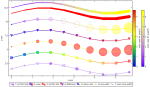Example (JKQTPlotter): Scatter Graph with Parametrized Symbols/Colors `JKQTPXYParametrizedScatterGraph`
C++-style QVector as plot data
modify scatter/points/line-graph properties by data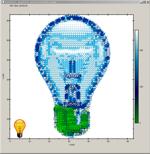Example (JKQTPlotter): Draw an Artistic Image with a Parametrized Scatter Graph `JKQTPXYParametrizedScatterGraph`
C++-style QVector as plot data
rectangular arrangement of scatters
generative computer graphics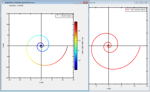Example (JKQTPlotter): Plotting Parametric Curves `JKQTPXYLineGraph` and `JKQTPXYParametrizedScatterGraph`
C++-style QVector as plot data
parametric curve plotting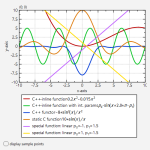Example (JKQTPlotter): Plotting Mathematical Functions as Line Graphs `JKQTPXFunctionLineGraph`
diretly plotting C/C++-functions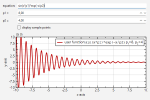Example (JKQTPlotter): Plotting Parsed Mathematical Functions as Line Graphs `JKQTPXParsedFunctionLineGraph`
plotting functions with the internal math equation parser/evaluator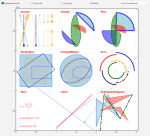Example (JKQTPlotter): Plotting Geometric Objects `JKQTPPlotObject`, `JKQTPGeoArc`, `JKQTPGeoLine`, `JKQTPGeoRectangle`, ...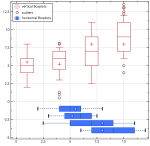Example (JKQTPlotter): Boxplots `JKQTPBoxplotVerticalGraph`, `JKQTPBoxplotHorizontalGraph`, ...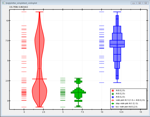Example (JKQTPlotter): Violin Plots `JKQTPViolinplotVerticalElement`, `JKQTPViolinplotHorizontalElement`, ...

## Styling the Plot, Keys, Axes, ...

Screenshot Description NotesExample (JKQTPlotter): Line Graph with Logarithmic y-axis `JKQTPXYLineGraph` and `JKQTPGeoText`
C++ vector of data
logarithmic axes and styling
plot line styles
internal LaTeX parser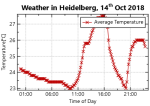Example (JKQTPlotter): Date/Time Axes `JKQTPXYLineGraph` and `JKQTPFilledVerticalRangeGraph`
C++ vector of data
date/time axes
plot min/max range graph
internal LaTeX parser
data from CSV files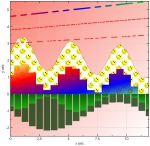Example (JKQTPlotter): Advanced Line and Fill Styling `JKQTPXYLineGraph`, `JKQTPSpecialLineHorizontalGraph` and `JKQTPBarVerticalGraph`
C++ vector of data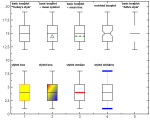Example (JKQTPlotter): Styling different aspects of boxplots Modifying different Aspects of the Styling of boxplots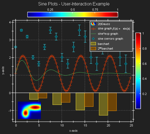Tutorial (JKQTPlotter): Styling a JKQtPlotter Modifying different Aspects of the Styling of JKQTPlotter

## Image data Plots

Screenshot Description Notes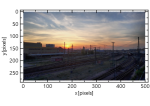Example (JKQTPlotter): QImage as a Graph `JKQTPImage`
`QImage` drawn onto a plot with arbitrary scaling
inverted coordinate axes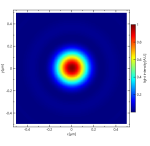Example (JKQTPlotter): Simple math image plot `JKQTPColumnMathImage`
image data copied from C-style row-major array into a single column of the internal datastore
Describes several options of the image plotting classes (different ways of color coding, what to do with data above/below the limits etc.)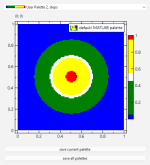Example (JKQTPlotter): Image Plots with Custom Palettes `JKQTPColumnMathImage`
user-defines palettes
palettes from files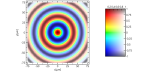Example (JKQTPlotter): Simple math image plot with modifier datat `JKQTPColumnMathImage`
image data copied from C-style row-major array into a single column of the internal datastore
Image is modified by a second image to display two data dimensions at the same time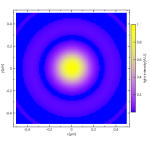Example (JKQTPlotter): Simple math image plot without use of central JKQTdatastore `JKQTPMathImage`
image data in a C-style row-major array, not using internal datastore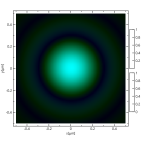Example (JKQTPlotter): Simple Math RGB/CMY Image Plot `JKQTPColumnRGBMathImage`
image data in a C-style row-major array, not using internal datastore
RGB/CMY color compositing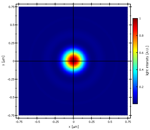Example (JKQTPlotter): Simple math image plot, showin a 1-channel OpenCV cv::Mat `JKQTPColumnMathImage`
image data copied from OpenCV cv::Mat-structure into a single column of the internal datastore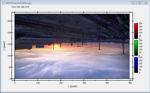Example (JKQTPlotter): Simple RGB image plot, showing a 3-channel OpenCV cv::Mat `JKQTPColumnRGBMathImage`
image data copied from OpenCV cv::Mat-structure into three columns of the internal datastore
inverted coordinate axes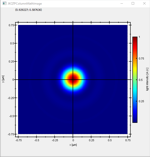Example (JKQTPlotter): Simple math image plot, showin a 1-channel CImg image `JKQTPColumnMathImage`
image data copied from CImg datastructure into a single column of the internal datastore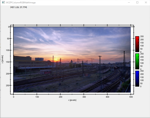Example (JKQTPlotter): Simple math image plot, showing a 3-channel CImg image `JKQTPColumnRGBMathImage`
image data copied from CImg datastructure into three columns of the internal datastore
inverted coordinate axes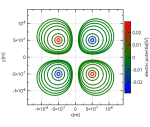Example (JKQTPlotter): Contour Plots `JKQTPColumnContourPlot`
image data edited inside JKQTPDatastore

## GUI Tools

Screenshot Description Notes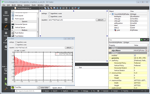Tutorial (JKQTPlotter): Using a JKQTPlotter inside a Qt User Interface Designer (UI) File using Qt Form Designer
parsed function plots (`JKQTPXParsedFunctionLineGraph`)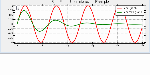Tutorial (JKQTPlotter): User-Interactions in JKQtPlotter different possibilities of user-interaction in JKQtPlotter

## Layout & Styling

Screenshot Description NotesExample (JKQTPlotter): Laying out Several Plots Combining plots in Qt Layouts
copy data from a `std::map` int the datastore
print plots/print previewTutorial (JKQTPlotter): Styling a JKQtPlotter Modifying different Aspects of the Styling of JKQTPlotterExample (JKQTPlotter): Image Plots with Custom Palettes `JKQTPColumnMathImage`
user-defines palettes
palettes from files

## Data Management & Statistics

Screenshot Description Notes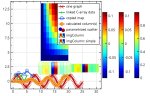Tutorial (JKQTPDatastore): Basic Usage of JKQTPDatastore Basic Data Management with JKQTPDatastore
Copying data into a JKQTPDatastore
Editing data inside a JKQTPDatastore
Editing Image Data in a JKQTPDatastore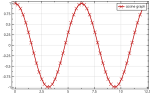Tutorial (JKQTPDatastore): Iterator-Based usage of JKQTPDatastore Iterator-based Data Management with JKQTPDatastore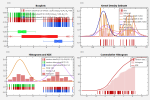Tutorial (JKQTPDatastore): Advanced 1-Dimensional Statistics with JKQTPDatastore Advanced 1-Dimensional Statistical Computation with JKQTPDatastore
using the internal statistics library (see Statistical Computations )
basic statistics (mean, standard deviation, ...)
boxplots
histograms
kernel density estimates (KDE)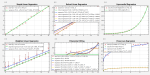Tutorial (JKQTPDatastore): Regression Analysis (with the Statistics Library) Advanced 1-Dimensional Statistical Computation with JKQTPDatastore
using the internal statistics library
Regression Analysis (with the Statistics Library)
robust regression (IRLS)
weighted regression
non-linear regression
polynomial fitting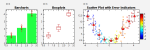Tutorial (JKQTPDatastore): 1-Dimensional Group Statistics with JKQTPDatastore Advanced 1-Dimensional Statistical Computation with JKQTPDatastore
grouped statistics
error indicators from data
boxplots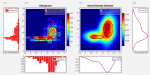Tutorial (JKQTPDatastore): Advanced 2-Dimensional Statistics with JKQTPDatastore Advanced 2-Dimensional Statistical Computation with JKQTPDatastore
using the internal statistics library (see Statistical Computations )
histograms
kernel density estimates (KDE)

## More Complex Examples

Screenshot Description NotesExample (JKQTPlotter): Laying out Several Plots Combining plots in Qt Layouts
copy data from a `std::map` int the datastore
print plots/print preview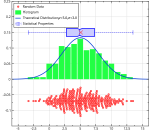Example (JKQTPlotter): Plotting a Statistical Distribution of Data Combines several different graphs to draw random values, their distribution and some statistical properties

## Tools and Special Features

Screenshot Description Notes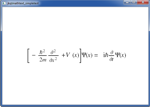Example (JKQTMathText): A simple usage example JKQTMathText
render LaTeX markup (Schrödinger's equation)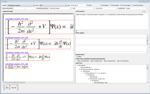Example (JKQTMathText): A test application JKQTMathText
render LaTeX markup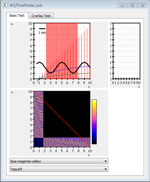Example (JKQTFastPlotter) JKQTFastPlotter

## CMake Build System

Screenshot Description NotesExample (JKQTPlotter): CMake Example explains how to link against JKQTPlotter with CMake